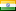CFD programs to solve simple problems in this project.

• Posted at : 5 months ago
• Post Similar Project
5000

Budget
3
Proposals
117
Views
Closed
Status
Skills Required

Posted By -

EEIndia
5.0
Projects Posted : 190
Projects Paid : 66
Services Purchased : 0
Total Spent :
4040
Feedbacks : 85 %

Project Details hide (-)

(1) Find out the equation of motion of the cylinder. The derivation procedure of the equation
must be included in the report.
(2) Develop a numerical method for predicting the vibration of the cylinder. The procedure of
deriving the FDM formula must be included in the report.
(3) Develop a MATLAB program for solving the problem. The MATLAB program code must be
included in the report.
(4) Keeping the damping coefficient of c = 100 N·m/s, conduct numerical simulations for KC=2
to 20 with an interval of 2 and find out the variation of the power with the KC number.
(5) Keeping the KC number to be constant of KC=10, conduct numerical simulations for c=0 to
2000 N·m/s with an interval of 50 and find the variation of the power with c.
(6) In the report, show the time histories of the vibration for all the calculated cases.
(7) Discuss how KC and c affects the power generation.